Tao, Y., and Li, P. (2016). "Analyzing the relationships between processing parameters and fractal dimension of void size on cross-sections of oriented strandboards," BioRes. 11(4), 9154-9161.

#### Abstract

Oriented strandboards (OSB) having various properties were constructed by varying processing parameters including strand thickness, strand length, and panel density. A calculation method was developed for analyzing the fractal dimension of void size (FDVS) on the cross-section of OSB samples based on a computer image processing technique and the fractal geometry theory. The results showed that The FDVS on the cross-section of OSB varied with different processing parameters. The FDVS decreased with strand thickness and increased with panel density, whereas the FDVS irregularly changed with strand length. Especially for panels with the same overall porosity, the FDVS was dependent on the internal structure. Therefore, the FDVS could be a useful additional parameter for characterizing the internal structure of OSB.

Analyzing the Relationships between Processing Parameters and Fractal Dimension of Void Size on Cross-Sections of Oriented Strandboards

Yubo Tao and Peng Li *

Oriented strandboards (OSB) having various properties were constructed by varying processing parameters including strand thickness, strand length, and panel density. A calculation method was developed for analyzing the fractal dimension of void size (FDVS) on the cross-section of OSB samples based on a computer image processing technique and the fractal geometry theory. The results showed that The FDVS on the cross-section of OSB varied with different processing parameters. The FDVS decreased with strand thickness and increased with panel density, whereas the FDVS irregularly changed with strand length. Especially for panels with the same overall porosity, the FDVS was dependent on the internal structure. Therefore, the FDVS could be a useful additional parameter for characterizing the internal structure of OSB.

Keywords: Fractal dimension; Porosity; Voids; Oriented Strandboards

Contact information: College of Material Science and Engineering, Northeast Forestry University, Harbin 150040, China; *Corresponding author: lptyb@aliyun.com

INTRODUCTION

Oriented strandboard (OSB) products are manufactured from wood strands with relatively small quantities of thermal-setting adhesive. Because of the nature of the mat-forming process, many voids are present in the cross-section of OSB. Previous studies indicate that the knowledge about void characteristics is useful for optimizing the manufacturing process and improving the final properties of the wood-strand composites (Suchsland and Xu 1989; Kamke and Wolcott 1991; Dai and Steiner 1993; Lang and Wolcott 1995; Zombori et al. 2003; Li et al. 2009). Most studies in this field has dealt with composite porosity only. The void structure of the porous materials can be significantly different even at the same overall porosity level, which can result in great deviations in heat and mass transfer during hot pressing, and, as a consequence, the performance properties of the final products (e.g., strength and modulus) can also be very different. Therefore, in addition to the overall porosity, new void characterization techniques are needed, which could contribute to the development of more realistic analytical models for OSB manufacturing.

Fractal theory is a non-Euclidean geometry theory that describes the irregularity and self-similarity in nature (Mandelbrot et al. 1984). By investigating the irregular shape and self-similarity of a natural object, the definition of space dimension can be extended. The so-called fractal dimension explains the randomness and self-similarity of fractal geometrical objects (Mandelbrot 1982). For materials with complex and irregular porous structure, the fractal theory provides an appropriate mathematical tool to study the relationship between void and density, on the one hand, and material performance (e.g., modulus and strength), on the other. Therefore, fractal geometry has been widely applied to study the internal structure morphology of a great variety of porous materials, including soil (Bird and Perrier 2003), concrete (Diamond 1999), and porous silica (Yamaguchi et al. 2008). In fractal theory, the Sierpinski carpet (Winslow 1985; Barnsley 1988; Li et al. 2013) can be applied to simulate the void structure.

The objective of this study was to develop a calculation method for analyzing the fractal dimension of void size (FDVS) on the cross-section of OSB, based on a computer image processing technique. The effect of OSB processing parameters on the fractal dimension of void size is presented and discussed.

EXPERIMENTAL

OSB Manufacturing Parameters

The following parameters were the same for all panels in the panel manufacturing process. Panel dimensions were 600 × 600 × 11 mm (length × width × thickness), with a random strand orientation and 6% to 8% moisture content. Aspen strands were manufactured in the laboratory with a width of 30 mm, a density of 400 kg m-3, and 4% MDI resin. The pressing temperature was 210 °C for 3 min. Each group (from 1 to 12) of panels was tested with three repetitions. Three key variables were chosen for this study: strand thickness, strand length, and panel density. The variables were changed in the ranges as summarized in Table 1.

Table 1. Processing Conditions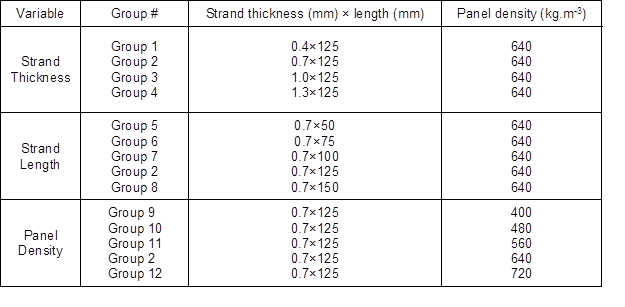Detecting Panel Voids using Image Processing

OSB panels were cut into 50 × 50 mm2 samples with very flat, clear edges. Every panel condition (group) had three repetitions. Each repetition had one set of eight 50 × 50 mm2samples for imaging and analyzing, i.e., 32 (4 × 8) edges for void measurements per panel condition. Thus, every panel condition (group) had 96 (32 ×3) edges (50 mm × 11mm) for void measurements.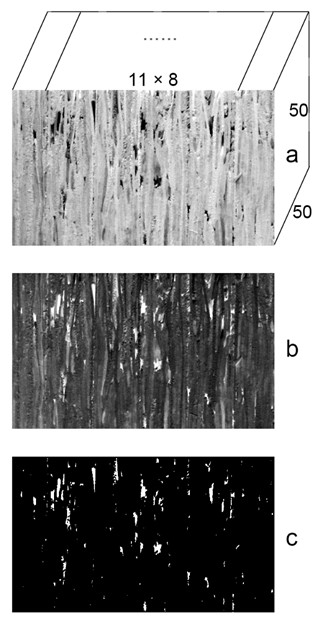Fig. 1. Processed image analysis procedure: (a) original image showing a typical cross-section photo of a set of eight 11×50×50 mm3 OSB samples; (b) complemented image; (c) image used to analyze the properties of the voids

The measurements involved two steps: digital photo imaging and image analysis. Images of the panel edges were digitally photographed using a high resolution CCD digital camera (Sony DSC-S70, Sony Inc. Japan). An image from the camera provided a precision level of 80 μm per pixel. The images were in digital RGB (2048 × 1536 × 8 bit) format. The images were then analyzed using the MATLAB software (MathWorks Inc., Natick, MA) image processing toolbox. All programs were written in the M-file format using MATLAB syntax. The major program steps included reading an image, identifying the voids, and characterizing the voids. Figure 1 shows the method of detecting panel voids. To visualize the voids, an original image (Fig. 1a) was first loaded to the MATLAB workspace using the imread function. The MATLAB software encoded the digital data into an array (two-dimensional array for a grayscale image). A complement of the image was conducted using the imcomplement function. In the output image, dark areas become lighter and light areas become darker. This step ensured that the pixels’ values for the voids were higher than those of the background, as illustrated in Fig. 1b. The complemented image was thresholded into a binary image, with the im2bw function at a level specified by the graythresh function. Tiny voids were then erased using the thestrel function, as shown in Fig. 1c. The obtained image was finally used to analyze the properties of the voids using the regionprops function, which included the area and porosity (the ratio of total void areas to the panel cross-section area) and this data was converted into a structured array (Li et al. 2007).

FDVS Calculation Method

The FDVS of OSB samples was determined based on the fractal geometry theory and previous void structure research on other porous materials. The “Sierpinski carpet model” (Winslow 1985; Barnsley 1988) was applied to simulate the fractal void size on cross-sections of OSB. Figure 2 shows the Sierpinski carpet approach. A square with side lengths equal to 1 was used as the initial element. Each side of the square was divided into a number of m equal parts (Fig. 2a). Thus, the initial square was divided into a number of small squares with an area of m2. A number of n small squares, with a side length equal to 1/m, were then randomly selected and removed from the initial square (Fig. 2b). The remaining number of small squares is equaled to m2n. The same operation was continued with the remaining small squares. As a result, the squares became smaller in size, while the quantity of squares increased (Fig. 2c, d). The black part of the graph represents the entity, while the white part of the graph represents the void. Figure 2, parts e, f, and g can be drawn using the same approach but different parameters (m and n). Especially, both Sierpinski carpet models Fig. 2b and 2f exhibit the same porosity; however, their void structures are different. The maximum void size in Fig. 2b and c was greater than that of Fig. 2f and g.

The FDVS (D) of Sierpinski carpet model is defined in Eq.1,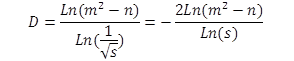(1)

where s is the area of minimum void.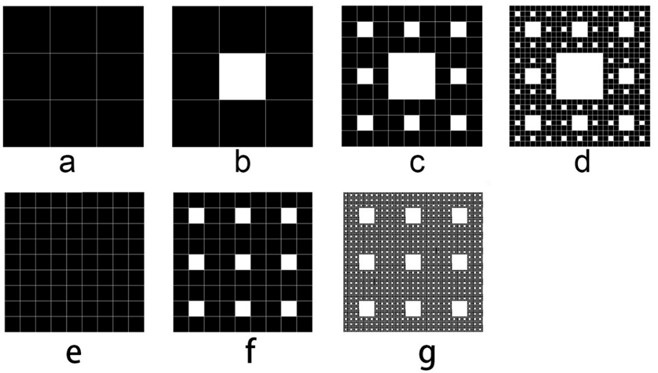Fig. 2. Formation of the Sierpinski carpet: (a) each side represents a number of m (m = 3) equal parts; (b) a number n (n = 1) of small squares were removed; (c) the same operation was continued based on (b); (d) the same operation is continued based on (c); (e) each side represents a number of m (m = 9) equal parts; (f) a number n (n = 9) of small squares were removed; and (g) the same operation was continued based on (f)

The FDVS (D) as represented in Fig. 2b, c, and d, is shown in Eq. 2: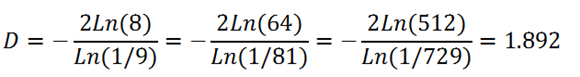(2)

The void data in Fig. 2a, b, c, and d are shown in Table 2. According to Table 2, a characteristics graph of the Sierpinski carpet (m=3, n=1) can be drawn, as shown in Fig. 3. Figure 3a demonstrates that smaller size voids have a higher quantity. Figure 3b shows the linear relationship between the logarithm of void area and logarithm of void quantity. In Fig. 3b, the slope of the linear regression was 0.946. According to the fractal theory, the FDVS of this Sierpinski carpet was 1.892 (0.946×2). Using the same calculation method, when m and n of the Sierpinski carpet model are equal to 9, as shown in Fig. 2f and 2g, the FDVS is equal to 1.934. Obviously, two kinds of Sierpinski carpet models (Fig. 2b and Fig. 2f) have a same porosity but different FDVS. Smaller void size can result in greater FDVS. And if the quantity of voids is zero (n =0), the fractal dimension of equaled 2.

Table 2. Parameters of the Sierpinski Carpet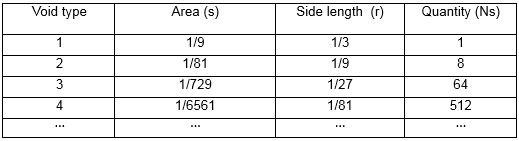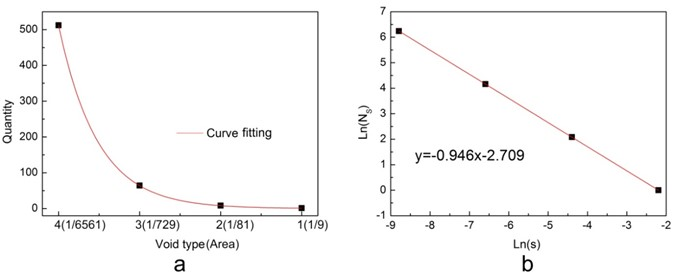Fig. 3. Characteristics graph of Sierpinski carpet model: (a) curve fitting between void type (area) and the quantity of voids; (b) natural logarithm relationship between the void area and the quantity

RESULTS AND DISCUSSION

FDVS Calculation of Panel Sample

According to the calculation method for the FDVS in the Sierpinski carpet and the data from the image analysis, the void characteristic graphs of the panel sample cross-section for every group can be drawn (Fig. 4 shows such graphs).

A program was written to automatically calculate the quantity of the voids under different area ranges. Figure 4a demonstrates that a smaller area void can cover a larger quantity. Figure 4b shows that the linear relationship between the logarithm of void area, the logarithm of void quantity, and the slope of the linear regression was 0.918. According to the Sierpinski carpet model, the FDVS was 1.836 (0.918×2). Compared with the exact fractal of the Sierpinski carpet, the void size of the panel cross-section was approximately fractal. The fractal dimension can be used to characterize the structure of the cross-section. The data obtained by this method are plotted in Figs. 5, 6, 7, and 8.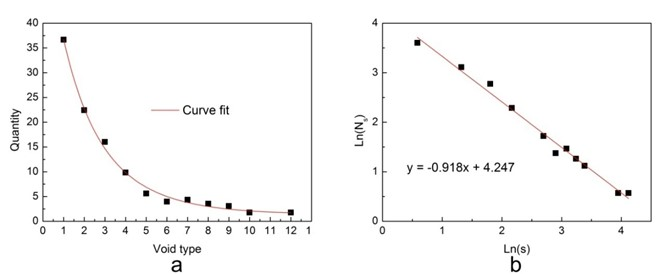Fig. 4. One of the void characteristics graphs of panel sample cross-section: (a) relationship between the void type and the quantity; (b) relationship between the void size and the quantity (slope = 0.918)

Relationship between Processing Parameters and FDVS

Figure 5 shows that at a given panel density and strand length, the FDVS decreased and the porosity increased with increasing strand thickness. Thicker strands lead to less uniformity in mat formation and more difficulty in mat consolidation; therefore, the porosity increased with increasing strand thickness. Higher porosity lead to lower FDVS.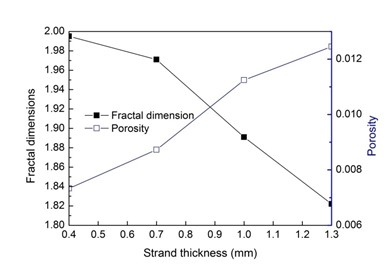Fig. 5. Dependency of FDVS and porosities on strand thickness (strand length 125 mm; panel density 640 kg.m-3)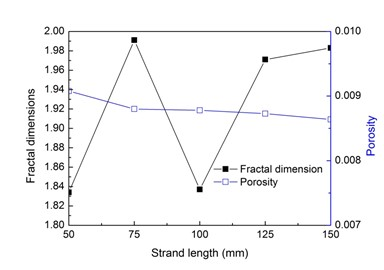Fig. 6. Dependency of FDVS and porosities on strand length (strand thickness 0.7 mm; panel density is 640 kg.m-3)

Figure 6 demonstrates that the difference in strand length (50 mm to 150 mm) has little impact on the porosity in contrast to the strand thickness (Fig. 5). In general, longer strand lengths result in slightly lower porosity (Li et al. 2009); however, the porosity is nearly the same. The data revealed an irregular relationship between the FDVS and the strand length. As the strand lengths increased from approximately 50 mm to 150 mm, the FDVS increased first before decreasing and then increased again. The lower FDVS appear to be at the strand lengths of 50 and 100 mm. The fractal theory could be used for explaining the reason of this phenomenon. As shown in Fig. 2b and f, both Sierpinski carpet models exhibit the same porosity, however, the FDVS are different. The maximum void size in Fig. 7b was greater than that of Fig. 7f. Accordingly, different strand lengths can lead to various internal void structures of the wood-strand composites. The internal void structures not only affect the porosity of the panel, but they also affect the distribution of void sizes. When OSB panels have different internal void structures at the same porosity level, greater FDVS values are represented for a smaller maximum void size and for more voids in the panels.

Figure 7 shows that the porosity decreased and the FDVS increased with an increase in the panel density. Understandably, higher density panels should have a lower porosity and a higher fractal dimension of the void size. The effect of panel density was more obvious than that of the strand length and width.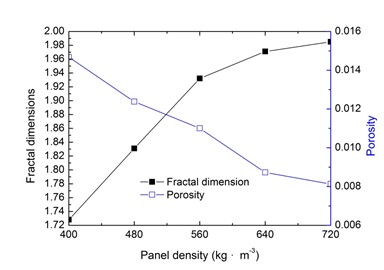Fig. 7. Dependency of FDVS and porosities on panel density (strand thickness 0.7 mm; strand length 125 mm)

CONCLUSIONS

1. A combination of image processing techniques and the fractal theory contributed to the void structure characterization on the cross-section of OSB.
2. The FDVS varied with different processing parameters. The FDVS decreased with strand thickness and increased with panel density, whereas the FDVS irregularly changed with strand length.
3. At same overall porosity level, the FDVS was dependent on the internal void structure. The FDVS was useful for describing structural differences in OSB, especially for panels with the same overall porosity, but different void structures.
4. The method for analyzing FDVS can be used for modeling fractal heat and mass transfer, resulting in more realistic data.

ACKNOWLEDGMENTS

This project was supported by the Fundamental Research Funds for the Central Universities of China (DL10CB02) and the Program for New Century Excellent Talents in University (NCET-13-0711). The authors would like to thank Sunguo Wang, Siguo Chen, and Zelong Li for their help and advice.

REFERENCES CITED

Barnsley, M. (1988). Fractals Everywhere, Academic Press, Cambridge, MA.

Bird, N. R. A., and Perrier, E. M. A. (2003). “The pore-solid fractal model of soil density scaling,” Eur. J. Soil Sci. 54(3), 467-476. DOI: 10.1046/j.1365-2389.2003.00481.x

Dai, C., and Steiner, P. R. (1993). “Compression behavior of randomly formed wood flake mats,” Wood Fiber Sci. 25(4), 349-358.

Diamond, S. (1999). “Aspects of concrete porosity revisited,” Cement Concrete Res. 29(8), 1181-1188. DOI: 10.1016/S0008-8846(99)00122-2

Kamke, F. A., and Wolcott, M. P. (1991). Fundamentals of flake board manufacture: Wood-moisture relationships,” Wood Sci. Technol. 25(1), 57-71.

Lang, M. E., and Wolcott, M. P. (1995). “Modeling the consolidation of wood-strand mat,” in: Mechanics of Cellulosic Materials, AMD-Vol. 209/MD-Vol. 60. American Soc. of Mechanical Engineers (ASME), New York.

Li, P., Dai, C., and Wang, S. (2009). “A simulation of void variation in wood-strand composites during consolidation,” Holzforschung 63(3), 357-361. DOI: 10.1515/HF.2009.044

Li, P., Wang, F., Chen, S., and Wang, S. (2007). “Application of image processing programs for OSB research,” J. Comput. Inform. Syst. 3(2), 733-739

Li, P., Wu, Q., and Tao, Y. (2013). “Fractal dimension analysis of void size in wood-strand composites based on X-ray computer tomography images,” Holzforschung 67(2), 177-182. DOI: 10.1515/hf-2012-0074

Mandelbrot, B. B. (1982). The Fractal Geometry of Nature, Freeman, New York, NY.

Mandelbrot, B. B., Passoja, D. E., Paullay, A. J. (1984). “Fractal character of fracture surface of metals,” Nature 308, 721-722. DOI: 10.1038/308721a0

Suchsland, O., and Xu, H. (1989). “A simulation of the horizontal density distribution in a flakeboard,” Forest Prod. J. 39(5), 29-33.

Winslow, D. N. (1985). “Fractal character in cement and concrete,” Cement Concrete Res. 15(10), 817-824.

Yamaguchi, D., Mayama, H., Koizumi, S., Tsujii, K., and Hashimoto, T. (2008). “Investigation of self-assembled fractal porous silica over a wide range of length scales using a combined small-angle scattering method,” Eur. Phys. J. B. 63(2), 153-163. DOI: 10.1140/epjb/e2008-00223-9

Zombori, B. G., Kamke, F. A., and Watson, L.T. (2003). “Simulation of the internal conditions during the hot-pressing process,” Wood Fiber Sci. 35(1), 2-23.

Article submitted: July 1, 2015; Peer review completed: Aug. 11, 2016; Revised version received and accepted: August 31, 2016; Published: September 12, 2016.

DOI: 10.15376/biores.11.4.9154-9161